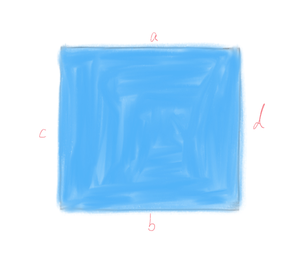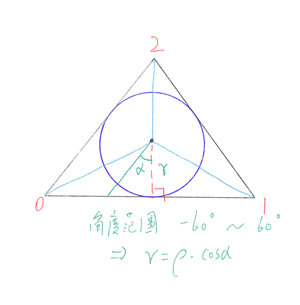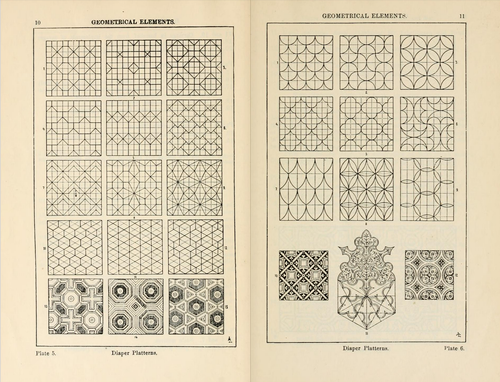## 常见形状

### 矩形### 正多边形$r = \rho \cdot cos\alpha \\ \Rightarrow \rho = \frac{r}{cos\alpha}$

### 极坐标

$\rho = \rho(\theta)$

$\rho$就是当前点距离中心点的长度；而$\theta$则是当前点相对于中心点的角度（弧度）；只要图形边缘满足上述特点即可使用极坐标进行绘制，如最常见的圆形，可以使用如下极坐标方程：

$\rho = a$

$a$是任意常数；

1. 叠加
2. 扣除

## 图案（patterns）

—— https://baike.baidu.com/item/图案/52183